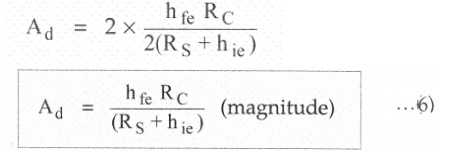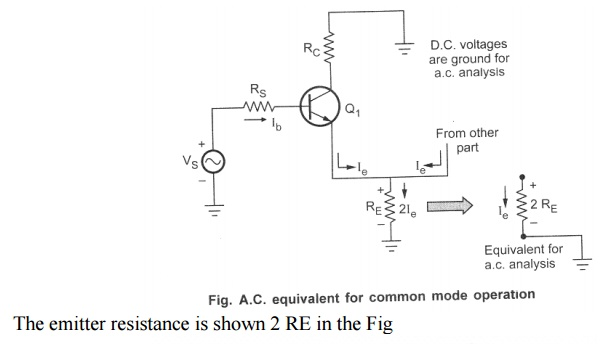Home | | Electronic Circuits I | A.C. Analysis of Differential Amplifier using h-Parameters

# A.C. Analysis of Differential Amplifier using h-Parameters

In the a.c. analysis, we will calculate the differential gain Ad, common mode gain A C, input resistance Ri and the output resistance R 0 of the differential amplifier circuit, using the h-parameters.

A.C. Analysis of Differential Amplifier using h-Parameters

In the a.c. analysis, we will calculate the differential gain Ad, common mode gain A C, input resistance Ri and the output resistance R 0 of the differential amplifier circuit, using the h-parameters.

1. Differential Gain (A d)

For the differential gain calculation, the two input signals must be different from each other. Let the two a.c. input signals be equal in magnitude but having 180" phase difference in between them. The magnitude of each a.c. input voltage V S1 and V S2beVs /2. The two a.c. emitter currents I e1 and I e2 are equal in magnitude and 180' out of phase. Hence they cancel each other to get resultant a.c. current through the emitter as zero. For the a.c. purposes emitter terminal can be grounded. The a.c. small signal differential amplifier circuit with grounded emitter terminal is shown in the Fig1 As the two transistors are matched, the a.c. equivalent circuit for the other transistor is identical to the one shown in the Fig..1. Thus the circuit can be analyzed by considering only one transistor. This is called as half circuit concept of analysis. The approximate hybrid model for the above circuit can be shown as in the Fig.2, neglecting hoe,The negative sign indicates the phase difference between input and output. Now two input signal magnitudes are VS /2 but they are opposite in polarity, as 180" out of phase.the expression for A d with balanced output changes asThis is the differential gain for balanced output dual input differential amplifier circuit.

2.  Common Mode Gain (A C)

Let the magnitude of both the a.c. input signals be VS and are in phase with each other. Hence the differential input Vd = 0 while the common mode input Vc is the average value of the two.But now both the emitter currents flows through R E in the Same direction. Hence the total current flowing through R E is 2I e. considering only one transistor, as in the FigStudy Material, Lecturing Notes, Assignment, Reference, Wiki description explanation, brief detail
Electronic Circuits : BJT Amplifiers : A.C. Analysis of Differential Amplifier using h-Parameters |